## Example Questions

### Example Question #1 : How To Find The Least Common Multiple

If a is the greatest common divisor of 64 and 14 and b is the least common multiple of 16 and 52 then a + b = ?

120

210

233

187

210

Explanation:

The greatest common divisor of 64 and 14 is 2, as found by the prime factorization of 64 and 14. The least common multiple of 16 and 52 is 208, which can be found by looking at the decimal when 52 is divided by 16. The remainder is 0.25, or 1/4 so the fourth multiple of 52 is 208, which is also divisible by 16.

### Example Question #1 : How To Find The Least Common Multiple

What is the least common multiple of 3, 4x, 5y, 6xy, and 10y?

60xy

25xy

60x2y2

30x2y2

30xy

60xy

Explanation:

Each of the numbers on the list must be able to "fit" (multiply evenly) into a larger number at the same time. I.e. the largest number (multiple) divided by any of the listed numbers will result in a whole number. For the coefficients, the maximum value is 10, and multiplying the highest two coefficients give us 60. Since 60 is divisible evenly by the lower values, we know that it is the least common multiple for the list. For the variables, both x and y will fit evenly into a theoretical number, "xy". We do not need an exponential version of this multiple as there are no exponents in the original list.

### Example Question #3 : How To Find The Least Common Multiple

What is the least common multiple of 45 and 60?

25

225

360

15

180

180

Explanation:

The least common multiple is the smallest number that is a multiple of all the numbers in the group.  Let's list some multiples of the two numbers and find the smallest number in common to both.

multiples of 45: 45, 90, 135, 180, 225, 270, ...

multiples of 60: 60, 120, 180, 240, 300, 360, ...

The smallest number in common is 180.

### Example Question #2 : How To Find The Least Common Multiple

What is the least common multiple of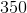and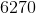?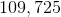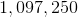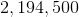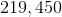Explanation:

Factor each of our values into prime factors:

350 = 2 * 52 * 7

6270= 2 * 3 * 5 * 11 * 19

To find the least common multiple, we must choose the larger exponent for each of the prime factors involved.  Therefore, we will select 2, 52, and 7 from 350 and 3, 11, and 19 from 6270.

Therefore, our least common multiple is 2 * 3 * 52 * 7 * 11 * 19 = 219,450.

Tired of practice problems?

Try live online GRE prep today.